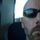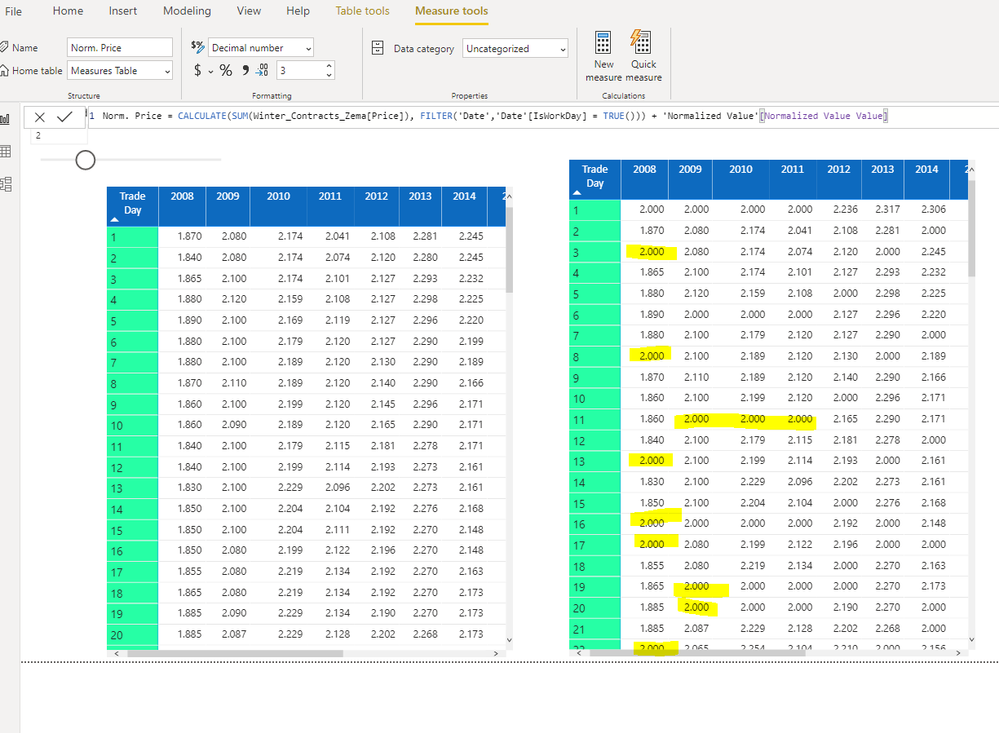cancel
Showing results for
Did you mean:Regular Visitor

## How do i create a date table ?

I was trying to create  a date table for filtering.. And ran across this DAX (below) ... thinking it looked useful.

However when I went to create the table and use it.. Didn't seem to work..

example

Date =

CALENDAR (DATE (2000, 1, 1), DATE (2025, 12, 31);

“DateAsInteger”; FORMAT ( [Date]; “YYYYMMDD” );

“Year”; YEAR ( [Date] );

“Monthnumber”; FORMAT ( [Date]; “MM” );

“YearMonthnumber”; FORMAT ( [Date]; “YYYY/MM” );

“YearMonthShort”; FORMAT ( [Date]; “YYYY/mmm” );

“MonthNameShort”; FORMAT ( [Date]; “mmm” );

“MonthNameLong”; FORMAT ( [Date]; “mmmm” );

“DayOfWeekNumber”; WEEKDAY ( [Date] );

“DayOfWeek”; FORMAT ( [Date]; “dddd” );

“DayOfWeekShort”; FORMAT ( [Date]; “dddd” );

“Quarter”; “Q” & FORMAT ( [Date]; “Q” );

“YearQuarter”; FORMAT ( [Date]; “YYYY” ) & “/Q” & FORMAT ( [Date]; “Q” )

)

I took it from this dude site.. .. Anyone have any suggestions for me on how I can quickly create the date table ..

28 REPLIES 28Anonymous
Not applicable

How do I create a column with number of working days in a year for the financial year? monday to friday.

Meaning if it starts in Nov 1

That 11/01/2020 Day Column starts with day 1 and ignores saturdays and sunday in the count

Is this possible?Super User

See this formula. You'll have to tweak it if you are not on a calendar year. The [Year] column would need to refer to a [Fiscal Year] for example.

``````WorksDay Count =
VAR CurrentDay = 'Date'[Date]
VAR CurrentYear = 'Date'[Year]
VAR WorkdayCount =
COUNTROWS(
FILTER(
ALL('Date'[Date],'Date'[IsWorkDay],'Date'[Year]),
'Date'[Date] <= CurrentDay
&& 'Date'[Year] = CurrentYear
&& 'Date'[IsWorkDay] = TRUE()
)
)
RETURN
WorkdayCount``````

It relies on another column called IsWorkday, which is:

``IsWorkDay = WEEKDAY('Date'[Date],2) < 6``With the IsWorkDay column, it is easy to count, filter, or determine if a day is a workday in visuals, measures, etc.

Creating a Dynamic Date Table in Power Query

Did my answers help arrive at a solution? Give it a kudos by clicking the Thumbs Up!

DAX is for Analysis. Power Query is for Data Modeling

Proud to be a Super User!

MCSA: BI ReportingAnonymous
Not applicable

Hi

Thanks that worked perfectly,However seems like the date table is not working connected with my data. Im trying to calculare the % change day over day. And i get wrong numbers when calculating with my date field on my data table, however when i use the date field from my data table then works but it doesnt exclude weekends as a result i get wrong values every monday of the dates.

Norm. Price = CALCULATE(sumX(Winter_Contracts_Zema,Winter_Contracts_Zema[Price]), FILTER('Date','Date'[IsWorkDay]= TRUE())) + 'Normalized Value'[Normalized Value Value]

Norm Price: Its my price field plus a variable [NormValue] = Value from 1 to 5 that changes the formula dynamically.

This is the DAX I'm using to calculate Last day value, which  gives me incorrect values for all mondays as it shows (2) which is the variable im adding to my price field.

However, using this DAX doesnt work when using date from date table. Norm Priced just shows 2 in all columns which is the Normalized value. and the DayoDay % still shows 2 for all mondays.

I also tried to add Is work day to my page filter but no luck

ANy ideas?I took @GilesWalker's solution (it's great) and modified and added some. The formulas are copy-and-paste ready too (no curvy quote format issues). Here's my preferred finished product:

https://sharepointlibrarian.com/2018/02/12/how-to-create-a-powerful-date-table-or-datekey-in-power-b...Skilled Sharer

@nchambe - thanks for the feedback and the great write up on your blog.

One change I have made to the formula for creating the table is similar to yours:

DateKey = CALENDAR(DATE(2017,07,01),DATE(YEAR(NOW())+1,06,30))

This way my end date will always move with the changing of calendar years and gives me the date to the end of the financial year.Skilled Sharer

@nchambe - thanks for the feedback and the great write up on your blog.

One change I have made to the formula for creating the table is similar to yours:

DateKey = CALENDAR(DATE(2017,07,01),DATE(YEAR(NOW())+1,06,30))

This way my end date will always move with the changing of calendar years and gives me the date to the end of the financial year.Anonymous
Not applicable

Sorry if I'm jumping on your thread for my own ends but I used the exact same formula from that website you've mentioned and wondered whether anyone was able to apply a filter on it. I just need to exclude the blanks from a selection slicer on my dashboard as it's taking up too much space. Anyone know how I could do this?Just swap all his semi colons with commas, and it works.

```Date =
CALENDAR (DATE(2000,1,1), DATE(2025,12,31)),
"DateAsInteger", FORMAT ( [Date], "YYYYMMDD" ),
"Year", YEAR ( [Date] ),
"Monthnumber", FORMAT ( [Date], "MM" ),
"YearMonthnumber", FORMAT ( [Date], "YYYY/MM" ),
"YearMonthShort", FORMAT ( [Date], "YYYY/mmm" ),
"MonthNameShort", FORMAT ( [Date], "mmm" ),
"MonthNameLong", FORMAT ( [Date], "mmmm" ),
"DayOfWeekNumber", WEEKDAY ( [Date] ),
"DayOfWeek", FORMAT ( [Date], "dddd" ),
"DayOfWeekShort", FORMAT ( [Date], "ddd" ),
"Quarter", "Q" & FORMAT ( [Date], "Q" ),
"YearQuarter", FORMAT ( [Date], "YYYY" ) & "/Q" & FORMAT ( [Date], "Q" )
)```Regular Visitor

TRy This

Date_New =
CALENDAR ( "1-jan-2010", "31-dec-2020" ),
"Date Key", FORMAT ( [Date], "YYYYMMDD" ),
"Year", YEAR ( [Date] ),
"Month number", FORMAT ( [Date], "MM" ),
"Year Month number", FORMAT ( [Date], "YYYY/MM" ),
"Year Month Short", FORMAT ( [Date], "YYYY/mmm" ),
"Month Name Short", FORMAT ( [Date], "mmm" ),
"Month Name Long", FORMAT ( [Date], "mmmm" ),
"Day of Week Number", WEEKDAY ( [Date] ),
"Day of Week", FORMAT ( [Date], "dddd" ),
"Day of Week Short", FORMAT ( [Date], "dddd" ),
"Quarter", "Q" & FORMAT ( [Date], "Q" ),
"Year Quarter", FORMAT ( [Date], "YYYY" ) & "/Q" & FORMAT ( [Date], "Q" )
)Regular Visitor

Thanks,

but its too long to understand & also DAX RUNDOWN also not working when calculate quarter for month which starting from APRIL.

Power BI default counts his FISCAL YEAR from JAN, Is there any option to change DAFAULT fiscal year in POWER BI.??Resident Rockstar

Hi

Try to createa new table using Power Query for master Date, The Power Query is

let
Source = (StartDate as date, EndDate as date, optional Culture as nullable text) as table =>
let
DayCount = Duration.Days(Duration.From(EndDate - StartDate)),
Source = List.Dates(StartDate,DayCount,#duration(1,0,0,0)),
TableFromList = Table.FromList(Source, Splitter.SplitByNothing()),
ChangedType = Table.TransformColumnTypes(TableFromList,{{"Column1", type date}}),
RenamedColumns = Table.RenameColumns(ChangedType,{{"Column1", "Date"}}),
InsertYear = Table.AddColumn(RenamedColumns, "Year", each Date.Year([Date])),
InsertQuarter = Table.AddColumn(InsertYear, "QuarterOfYear", each Date.QuarterOfYear([Date])),
InsertMonth = Table.AddColumn(InsertQuarter, "MonthOfYear", each Date.Month([Date])),
InsertDay = Table.AddColumn(InsertMonth, "DayOfMonth", each Date.Day([Date])),
InsertDayInt = Table.AddColumn(InsertDay, "DateInt", each [Year] * 10000 + [MonthOfYear] * 100 + [DayOfMonth]),
InsertMonthName = Table.AddColumn(InsertDayInt, "MonthName", each Date.ToText([Date], "MMMM", Culture), type text),
InsertCalendarMonth = Table.AddColumn(InsertMonthName, "MonthInCalendar", each (try(Text.Range([MonthName],0,3)) otherwise [MonthName]) & "'" & Text.Range(Number.ToText([Year]),2,2)),
InsertCalendarQtr = Table.AddColumn(InsertCalendarMonth, "QuarterInCalendar", each "Q" & Number.ToText([QuarterOfYear]) & " " & Number.ToText([Year])),
InsertDayWeek = Table.AddColumn(InsertCalendarQtr, "DayInWeek", each Date.DayOfWeek([Date])),
InsertDayName = Table.AddColumn(InsertDayWeek, "DayOfWeekName", each Date.ToText([Date], "dddd", Culture), type text),
InsertWeekEnding = Table.AddColumn(InsertDayName, "WeekEnding", each Date.EndOfWeek([Date]), type date),
InsertWeekStarting = Table.AddColumn(InsertWeekEnding, "WeekStart", each Date.StartOfWeek([Date]), type date)
in
InsertWeekStarting,
#"Invoked FunctionSource" = Source(#date(2016, 1, 1), #date(2016, 12, 31), null),
#"Changed Type" = Table.TransformColumnTypes(#"Invoked FunctionSource",{{"MonthOfYear", Int64.Type}, {"QuarterOfYear", Int64.Type}, {"DayOfMonth", Int64.Type}, {"DayInWeek", Int64.Type}}),
#"Duplicated Column" = Table.DuplicateColumn(#"Changed Type", "Date", "Date - Copy"),
#"Calculated Week of Year" = Table.TransformColumns(#"Duplicated Column",{{"Date - Copy", Date.WeekOfYear}}),
#"Renamed Columns" = Table.RenameColumns(#"Calculated Week of Year",{{"Date - Copy", "WeekofMonth"}}),
#"Changed Type1" = Table.TransformColumnTypes(#"Renamed Columns",{{"Year", Int64.Type}, {"DateInt", Int64.Type}, {"MonthInCalendar", type text}, {"QuarterInCalendar", type text}}),
#"Filtered Rows" = Table.SelectRows(#"Added Custom", each true),
#"Changed Type2" = Table.TransformColumnTypes(#"Filtered Rows",{{"Sorting Order", Int64.Type}})
in
#"Changed Type2"

It will help uSkilled Sharer

@sbowles The way I create a date table is as follows:

Click the insert new table button on the ribbon and copy in below:

DateKey = CALENDAR(DATE(2012,01,01),DATE(2017,06,30))

Then I add in a new column from the ribbon for each of the following:

Year = YEAR(DateKey[Date])
Month number = MONTH(DateKey[Date])
Month = FORMAT(DateKey[Date],"MMM")
Day = FORMAT(DateKey[Date],"ddd")
Week = WEEKNUM(DateKey[Date],1)
Quarter = "Q" & ROUNDUP(MONTH(DateKey[Date])/3,0)
MonthY = FORMAT(DateKey[Date],"MMM")&" " &DateKey[Year]
Day number = DAY(DateKey[Date])
Financial year = IF(DateKey[Month number]>6,DateKey[Year]+1,DateKey[Year])
Financial week = IF(DateKey[Month number]>6,DateKey[Week]-26,DateKey[Week]+26)
Total days = DAY(DATE(DateKey[Year],DateKey[Month number]+1,1)-1)
Financial month number = IF((DateKey[Month number]-6)<=0,DateKey[Month number]+6,DateKey[Month number]-6)
Financial month = FORMAT(DateKey[Date],"MMM")
Index = CALCULATE(COUNT(DateKey[Date]),ALL(DateKey[Date]),FILTER(DateKey,DateKey[Date]<=EARLIER(DateKey[Date])))
Monthy number = DateKey[Year]&DateKey[Month number]

I also include the following measures:

Measures
Current financial week = IF(WEEKNUM(NOW())-26<=0,WEEKNUM(NOW())+26,WEEKNUM(NOW())-26)
Current financial month = IF(MONTH(NOW())-6<=0,MONTH(NOW())+6,MONTH(NOW())-6)
Today = DATE(YEAR(NOW()),MONTH(NOW()),DAY(NOW()))

Then I can use these rolling filters:

Other formula
+/- 1 months = IF(AND(DateKey[Financial month number]>=[Current financial month]-1,DateKey[Financial month number]<=[Current financial month]),1,0)
Last 30 day = IF(AND(DateKey[Date]>=[Today]-30,DateKey[Date]<=[Today]),1,0)
Last week + two weeks = IF(AND(DateKey[Financial week]>=[Current financial week]-1,DateKey[Financial week]<=[Current financial week]+2),1,0)
Last 14 days = IF(AND(DateKey[Date]>=[Today]-14,DateKey[Date]<=[Today]),1,0)
+/- 30 days = IF(AND(DateKey[Date]>=[Today]-30,DateKey[Date]<=[Today]+30),1,0)

Hope this helps.

GilesThanks for this tip, very helpful!

I found that

`Index = CALCULATE(COUNT(DateKey[Date]),ALL(DateKey[Date]),FILTER(DateKey,DateKey[Date]<=EARLIER(DateKey[Date])))`

This calculated column will show #ERROR after I marked the [Date] column into the Date Table, so I changed the formula to:

```Index =
-DATEDIFF(
DateKey[Date],
CALCULATE(
MIN(DateKey[Date]),
ALL(DateKey)
),
DAY
) + 1```Helper I

I like your approach would this cover me for oct to october finicial year.

Also how would I filter based on last years then using this table in a measure I want to show last years previous sales?Anonymous
Not applicable

Hi @GilesWalker, Thanks for all the Dax formulas.

I have created Datekey table and all the other coloums, and created relationship with my flattable in powr bi desktop. But, when I am am trying to show data week wise, using week coloumn it is showing blank in X-axis.

My report requirment to show  last four week of sales using storewise and category wise.

Please advice me for better logicFrequent Visitor

This is a little out of scope here, but is there a way to create this table to dynamically update and add a new day?  For example, if I use the caledar(date(),date()) I'm obviously closing it with a specific date.  Any way to make it where it continues and does not have to be updated manually in the future?Helper II

@mpm000 Assuming you haven't already figured this out or found the answer in another thread, the following formula should auto-update based on the current date. (Note my time table starts Jan 2000.)

DateKey = CALENDAR( DATE(2000,01,01) , DATE(YEAR(NOW()) , MONTH(NOW()) , DAY(NOW()) ))Frequent Visitor

This is a little out of scope here, but is there a way to create this table to dynamically update and add a new day?  For example, if I use the caledar(date(),date()) I'm obviously closing it with a specific date.  Any way to make it where it continues and does not have to be updated manually in the future?Regular Visitor

we can't calculate our fiscal year from this formula

Quarter = "Q" & ROUNDUP(MONTH(DateKey[Date])/3,0)

My fiscal year start from April & end with March

Date Range = "Datekey = CALENDAR(DATE(2014, 04, 01),DATE(2017, 03, 31))"

ThanksAnonymous
Not applicable